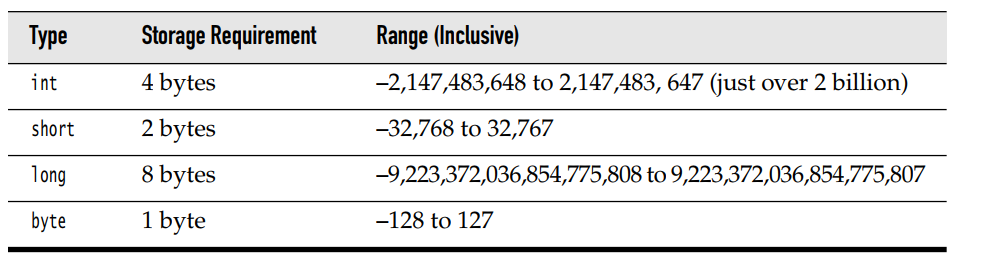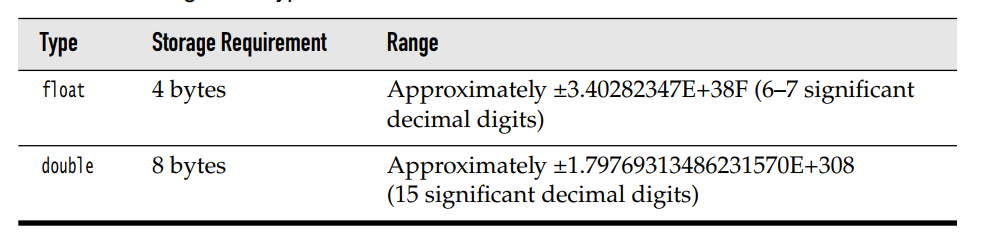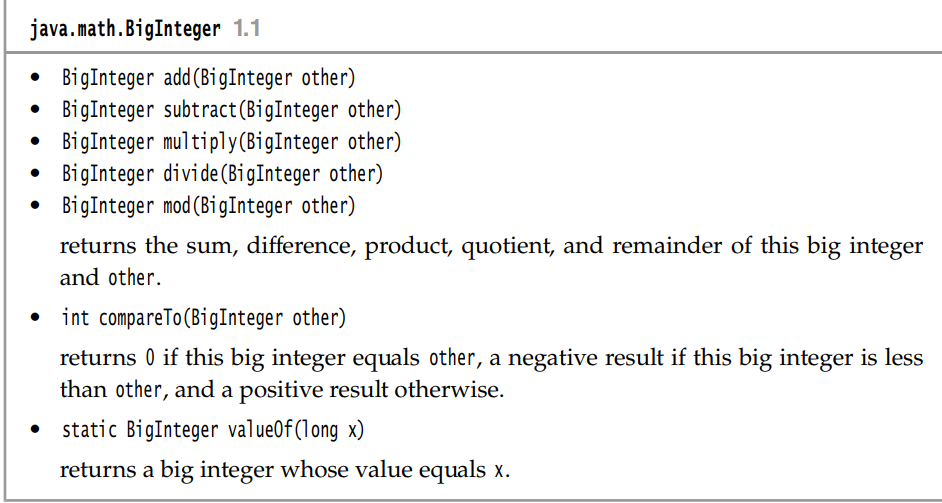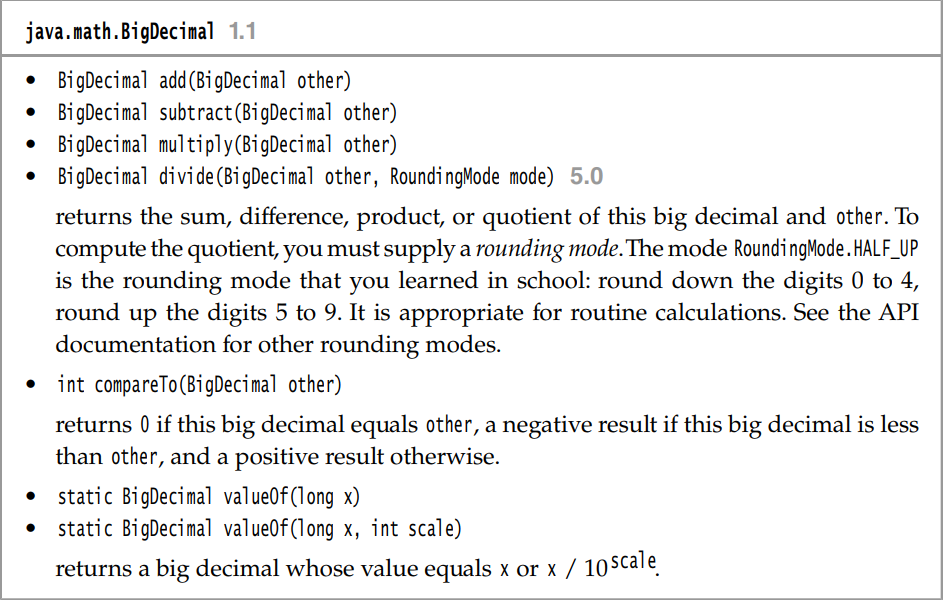• ## JAVA高精度计算

千次阅读 2019-05-08 20:25:39
高精度计算 用cpp实现较为复杂 但对于java 的Biginteger 和 Big Decimal来说就容易许多了。 先来一道题： http://codeforces.com/gym/101848/problem/A 总结一下各种类型的精度： 对于超过18位的运算，及时long ...

高精度计算 用cpp实现较为复杂 但对于java 的Biginteger 和 Big Decimal来说就容易许多了。

先来一道题： http://codeforces.com/gym/101848/problem/A
总结一下各种类型的精度：对于超过18位的运算，及时long 也不能精确表达式。（在cpp中 unsign long 是long 的两倍）
好在java中有两个 Big Numbers
理论上来说，内存有多大，这个数就能有多大。
BigInteger 和 BigInteger 的相关方法：两者的相互转化：
//BigDecimal to BigInteger
BigDecimal x1 = in.nextBigDecimal();
BigInteger xx1 = x1.toBigInteger();

//BigInteger to BigDecimal
BigDecimal result1 = new BigDecimal(xx1.toString());

题目的代码


import java.math.*;
import java.util.Scanner;

public class Test1{
public static void main(String arg[]){

Scanner in = new Scanner(System.in);
BigDecimal x1 = in.nextBigDecimal();
BigDecimal x2 = in.nextBigDecimal();
in.close();

BigDecimal xx = BigDecimal.valueOf(1e9);
x1=x1.multiply(xx);
x2=x2.multiply(xx);

BigInteger xx1 = x1.toBigInteger();
BigInteger xx2 = x2.toBigInteger();

BigInteger result = xx1.mod(xx2);

BigDecimal result1 = new BigDecimal(result.toString());
result1 = result1.divide(new BigDecimal(1e9));

System.out.printf("%.9f\n",result1);
}
}

展开全文• Java高精度计算Java高精度计算Java高精度计算Java高精度计算Java高精度计算Java高精度计算Java高精度计算Java高精度计算Java高精度计算Java高精度计算Java高精度计算Java高精度计算
• ## Java高精度计算

千次阅读 2018-05-15 19:47:30
代码如下：import java.util.Scanner;import java.math.BigDecimal;public class Main{ public static void main(String[] args) { Scanner cin = new Scanner(System.in); BigDecimal a = cin....

代码如下：

import java.util.Scanner;
import java.math.BigDecimal;

public class Main{

public static void main(String[] args) {

Scanner cin = new Scanner(System.in);

BigDecimal a = cin.nextBigDecimal();
BigDecimal b = cin.nextBigDecimal();

//高精度浮点数相加
//高精度浮点数相减
System.out.println(a.subtract(b));
//高精度浮点数相乘
System.out.println(a.multiply(b));
//高精度浮点数相除
System.out.println(a.divide(b));
//高精度浮点数取模
System.out.println(a.remainder(b));
//高精度浮点数取模并保留小数
System.out.printf("%.9f",a.remainder(b));
}
}


展开全文• Java高精度计算文件，很好用！！！非原创，特此向代码原作者致敬
• 主要为大家详细介绍了java精度计算代码，java指定精确小数位，具有一定的参考价值，感兴趣的小伙伴们可以参考一下
• 关于java高精度计算的问题。包含加、减、乘、除等运算
• ## Java 使用BigDecimal类处理高精度计算

万次阅读 多人点赞 2016-08-25 17:26:04
Javajava.math包中提供的API类BigDecimal，用来对超过16位有效位的数进行...一般情况下，对于那些不需要准确计算精度的数字，我们可以直接使用Float和Double处理，但是Double.valueOf(String) 和Float.valueOf(String
Java在java.math包中提供的API类BigDecimal，用来对超过16位有效位的数进行精确的运算。双精度浮点型变量double可以处理16位有效数，但在实际应用中，可能需要对更大或者更小的数进行运算和处理。一般情况下，对于那些不需要准确计算精度的数字，我们可以直接使用Float和Double处理，但是Double.valueOf(String) 和Float.valueOf(String)会丢失精度。所以开发中，如果我们需要精确计算的结果，则必须使用BigDecimal类来操作啦！
（一）BigDecimal类的常用的几个构造方法
BigDecimal(int)：将int表示形式转换为BigDecimal对象BigDecimal(String) ：将字符串表示形式转换为BigDecimal对象BigDecimal(double)：将double表示形式转换为BigDecimal对象
（二）BigDecimal类的常用方法
下面分享一个用于高精确处理常用的数学运算类
package com.per.test;

import java.math.BigDecimal;

/**
* 用于高精确处理常用的数学运算
* Created by lijuan on 2016/8/27.
*/
public class ArithmeticUtils {
//默认除法运算精度
private static final int DEF_DIV_SCALE = 10;

/**
* 提供精确的加法运算
*
* @param v1 被加数
* @param v2 加数
* @return 两个参数的和
*/

public static double add(double v1, double v2) {
BigDecimal b1 = new BigDecimal(Double.toString(v1));
BigDecimal b2 = new BigDecimal(Double.toString(v2));
}

/**
* 提供精确的加法运算
*
* @param v1 被加数
* @param v2 加数
* @return 两个参数的和
*/
public static BigDecimal add(String v1, String v2) {
BigDecimal b1 = new BigDecimal(v1);
BigDecimal b2 = new BigDecimal(v2);
}

/**
* 提供精确的加法运算
*
* @param v1    被加数
* @param v2    加数
* @param scale 保留scale 位小数
* @return 两个参数的和
*/
public static String add(String v1, String v2, int scale) {
if (scale < 0) {
throw new IllegalArgumentException(
"The scale must be a positive integer or zero");
}
BigDecimal b1 = new BigDecimal(v1);
BigDecimal b2 = new BigDecimal(v2);
}

/**
* 提供精确的减法运算
*
* @param v1 被减数
* @param v2 减数
* @return 两个参数的差
*/
public static double sub(double v1, double v2) {
BigDecimal b1 = new BigDecimal(Double.toString(v1));
BigDecimal b2 = new BigDecimal(Double.toString(v2));
return b1.subtract(b2).doubleValue();
}

/**
* 提供精确的减法运算。
*
* @param v1 被减数
* @param v2 减数
* @return 两个参数的差
*/
public static BigDecimal sub(String v1, String v2) {
BigDecimal b1 = new BigDecimal(v1);
BigDecimal b2 = new BigDecimal(v2);
return b1.subtract(b2);
}

/**
* 提供精确的减法运算
*
* @param v1    被减数
* @param v2    减数
* @param scale 保留scale 位小数
* @return 两个参数的差
*/
public static String sub(String v1, String v2, int scale) {
if (scale < 0) {
throw new IllegalArgumentException(
"The scale must be a positive integer or zero");
}
BigDecimal b1 = new BigDecimal(v1);
BigDecimal b2 = new BigDecimal(v2);
return b1.subtract(b2).setScale(scale, BigDecimal.ROUND_HALF_UP).toString();
}

/**
* 提供精确的乘法运算
*
* @param v1 被乘数
* @param v2 乘数
* @return 两个参数的积
*/
public static double mul(double v1, double v2) {
BigDecimal b1 = new BigDecimal(Double.toString(v1));
BigDecimal b2 = new BigDecimal(Double.toString(v2));
return b1.multiply(b2).doubleValue();
}

/**
* 提供精确的乘法运算
*
* @param v1 被乘数
* @param v2 乘数
* @return 两个参数的积
*/
public static BigDecimal mul(String v1, String v2) {
BigDecimal b1 = new BigDecimal(v1);
BigDecimal b2 = new BigDecimal(v2);
return b1.multiply(b2);
}

/**
* 提供精确的乘法运算
*
* @param v1    被乘数
* @param v2    乘数
* @param scale 保留scale 位小数
* @return 两个参数的积
*/
public static double mul(double v1, double v2, int scale) {
BigDecimal b1 = new BigDecimal(Double.toString(v1));
BigDecimal b2 = new BigDecimal(Double.toString(v2));
return round(b1.multiply(b2).doubleValue(), scale);
}

/**
* 提供精确的乘法运算
*
* @param v1    被乘数
* @param v2    乘数
* @param scale 保留scale 位小数
* @return 两个参数的积
*/
public static String mul(String v1, String v2, int scale) {
if (scale < 0) {
throw new IllegalArgumentException(
"The scale must be a positive integer or zero");
}
BigDecimal b1 = new BigDecimal(v1);
BigDecimal b2 = new BigDecimal(v2);
return b1.multiply(b2).setScale(scale, BigDecimal.ROUND_HALF_UP).toString();
}

/**
* 提供（相对）精确的除法运算，当发生除不尽的情况时，精确到
* 小数点以后10位，以后的数字四舍五入
*
* @param v1 被除数
* @param v2 除数
* @return 两个参数的商
*/

public static double div(double v1, double v2) {
return div(v1, v2, DEF_DIV_SCALE);
}

/**
* 提供（相对）精确的除法运算。当发生除不尽的情况时，由scale参数指
* 定精度，以后的数字四舍五入
*
* @param v1    被除数
* @param v2    除数
* @param scale 表示表示需要精确到小数点以后几位。
* @return 两个参数的商
*/
public static double div(double v1, double v2, int scale) {
if (scale < 0) {
throw new IllegalArgumentException("The scale must be a positive integer or zero");
}
BigDecimal b1 = new BigDecimal(Double.toString(v1));
BigDecimal b2 = new BigDecimal(Double.toString(v2));
return b1.divide(b2, scale, BigDecimal.ROUND_HALF_UP).doubleValue();
}

/**
* 提供（相对）精确的除法运算。当发生除不尽的情况时，由scale参数指
* 定精度，以后的数字四舍五入
*
* @param v1    被除数
* @param v2    除数
* @param scale 表示需要精确到小数点以后几位
* @return 两个参数的商
*/
public static String div(String v1, String v2, int scale) {
if (scale < 0) {
throw new IllegalArgumentException("The scale must be a positive integer or zero");
}
BigDecimal b1 = new BigDecimal(v1);
BigDecimal b2 = new BigDecimal(v1);
return b1.divide(b2, scale, BigDecimal.ROUND_HALF_UP).toString();
}

/**
* 提供精确的小数位四舍五入处理
*
* @param v     需要四舍五入的数字
* @param scale 小数点后保留几位
* @return 四舍五入后的结果
*/
public static double round(double v, int scale) {
if (scale < 0) {
throw new IllegalArgumentException("The scale must be a positive integer or zero");
}
BigDecimal b = new BigDecimal(Double.toString(v));
return b.setScale(scale, BigDecimal.ROUND_HALF_UP).doubleValue();
}

/**
* 提供精确的小数位四舍五入处理
*
* @param v     需要四舍五入的数字
* @param scale 小数点后保留几位
* @return 四舍五入后的结果
*/
public static String round(String v, int scale) {
if (scale < 0) {
throw new IllegalArgumentException(
"The scale must be a positive integer or zero");
}
BigDecimal b = new BigDecimal(v);
return b.setScale(scale, BigDecimal.ROUND_HALF_UP).toString();
}

/**
* 取余数
*
* @param v1    被除数
* @param v2    除数
* @param scale 小数点后保留几位
* @return 余数
*/
public static String remainder(String v1, String v2, int scale) {
if (scale < 0) {
throw new IllegalArgumentException(
"The scale must be a positive integer or zero");
}
BigDecimal b1 = new BigDecimal(v1);
BigDecimal b2 = new BigDecimal(v2);
return b1.remainder(b2).setScale(scale, BigDecimal.ROUND_HALF_UP).toString();
}

/**
* 取余数  BigDecimal
*
* @param v1    被除数
* @param v2    除数
* @param scale 小数点后保留几位
* @return 余数
*/
public static BigDecimal remainder(BigDecimal v1, BigDecimal v2, int scale) {
if (scale < 0) {
throw new IllegalArgumentException(
"The scale must be a positive integer or zero");
}
return v1.remainder(v2).setScale(scale, BigDecimal.ROUND_HALF_UP);
}

/**
* 比较大小
*
* @param v1 被比较数
* @param v2 比较数
* @return 如果v1 大于v2 则 返回true 否则false
*/
public static boolean compare(String v1, String v2) {
BigDecimal b1 = new BigDecimal(v1);
BigDecimal b2 = new BigDecimal(v2);
int bj = b1.compareTo(b2);
boolean res;
if (bj > 0)
res = true;
else
res = false;
return res;
}
}

好了，本篇文章就这样子啦，存在不对的地方还望指导，感谢^_^
参考文献：  java 处理高精度计算
展开全文string BigDecimal 数学运算
• BigDecimal 是标准的类，在编译器中不需要特殊支持，它可以表示任意精度的小数，并对它们进行计算。 用于加、减、乘和除的方法给 BigDecimal 值提供了算术运算。由于 BigDecimal 对象是不可变的，这些方法中的每...
首先看如下代码示例：
System.out.println(0.05 + 0.01);
System.out.println(0.05 - 0.03);
System.out.println(1.025 * 100);
System.out.println(305.1 / 1000);
输出结果为：
0.060000000000000005
0.020000000000000004
102.49999999999999
0.30510000000000004
Java语言支持两种基本的浮点类型：float和double，以及与它们对应的包装类Float和Double。它们都依据IEEE 754 标准，该标准为 32 位浮点和 64 位双精度浮点二进制小数定义了二进制标准。
IEEE 754 用科学记数法以底数为 2 的小数来表示浮点数。IEEE 浮点数用 1 位表示数字的符号，用 8 位来表示指数，用 23 位来表示尾数，即小数部分，作为有符号整数的指数可以有正负之分，小数部分用二进制（底数 2）小数来表示
不要用浮点值表示精确值
一些非整数值（如几美元和几美分这样的小数）需要很精确。浮点数不是精确值，所以使用它们会导致舍入误差。因此，使用浮点数来试图表示象货币量这样的精确数量不是一个好的想法。使用浮点数来进行美元和美分计算会得到灾难性的后果。浮点数最好用来表示象测量值这类数值，这类值从一开始就不怎么精确。
使用BigDecimal
从 JDK 1.3 起，Java 开发人员就有了另一种数值表示法来表示非整数： BigDecimal 。 BigDecimal 是标准的类，在编译器中不需要特殊支持，它可以表示任意精度的小数，并对它们进行计算。
用于加、减、乘和除的方法给 BigDecimal 值提供了算术运算。由于 BigDecimal 对象是不可变的，这些方法中的每一个都会产生新的 BigDecimal 对象。因此，因为创建对象的开销， BigDecimal 不适合于大量的数学计算，但设计它的目的是用来精确地表示小数。如果您正在寻找一种能精确表示如货币量这样的数值，则 BigDecimal 可以很好地胜任该任务。
构造 BigDecimal 数
对于 BigDecimal ，有几个可用的构造函数。其中一个构造函数以双精度浮点数作为输入，另一个以整数和换算因子作为输入，还有一个以小数的 String 表示作为输入。要小心使用 BigDecimal(double) 构造函数，因为如果不了解它，会在计算过程中产生舍入误差。请使用基于整数或 String 的构造函数。
public class Test {
public static void main(String[] args) {
// 以双精度浮点数进行构造
BigDecimal bd1 = new BigDecimal(0.5);
BigDecimal bd2 = new BigDecimal(0.1);

// 以String类型进行构造
BigDecimal bd3 = new BigDecimal("0.5");
BigDecimal bd4 = new BigDecimal("0.1");
}
}
输出结果为：
0.6000000000000000055511151231257827021181583404541015625
0.6
上面代码分别以
BigDecimal(double val)
BigDecimal(String val)
不同的方式进行构造 BigDecimal 数，输出的结果是不一样的。
回到最开始的示例，提供工具类进行精确的浮点数运算，包括加减乘除和四舍五入。
import java.math.BigDecimal;

public class ArithUtil {
private static final int DEF_DIV_SCALE = 6; // 默认除法运算精度

/**
* 提供精确的加法运算。
*
* @param v1 被加数
* @param v2 加数
* @return 两个参数的和
*/
public static double add(double v1, double v2) {
BigDecimal b1 = new BigDecimal(Double.toString(v1));
BigDecimal b2 = new BigDecimal(Double.toString(v2));

}

/**
* 提供精确的减法运算。
*
* @param v1 被减数
* @param v2 减数
* @return 两个参数的差
*/
public static double sub(double v1, double v2) {
BigDecimal b1 = new BigDecimal(Double.toString(v1));
BigDecimal b2 = new BigDecimal(Double.toString(v2));

return b1.subtract(b2).doubleValue();
}

/**
* 提供精确的乘法运算。
*
* @param v1 被乘数
* @param v2 乘数
* @return 两个参数的积
*/
public static double mul(double v1, double v2) {
BigDecimal b1 = new BigDecimal(Double.toString(v1));
BigDecimal b2 = new BigDecimal(Double.toString(v2));

return b1.multiply(b2).doubleValue();
}

/**
* 提供（相对）精确的除法运算，当发生除不尽的情况时，精确到 小数点以后10位，以后的数字四舍五入。
*
* @param v1 被除数
* @param v2 除数
* @return 两个参数的商
*/
public static double div(double v1, double v2) {
return div(v1, v2, DEF_DIV_SCALE);
}

/**
* 提供（相对）精确的除法运算。当发生除不尽的情况时，由scale参数指 定精度，以后的数字四舍五入。
*
* @param v1 被除数
* @param v2 除数
* @param scale  表示表示需要精确到小数点以后几位。
* @return 两个参数的商
*/
public static double div(double v1, double v2, int scale) {
if (scale < 0) {
throw new IllegalArgumentException(
"The scale must be a positive integer or zero");
}

BigDecimal b1 = new BigDecimal(Double.toString(v1));
BigDecimal b2 = new BigDecimal(Double.toString(v2));

return b1.divide(b2, scale, BigDecimal.ROUND_HALF_UP).doubleValue();
}

/**
* 提供精确的小数位四舍五入处理。
*
* @param v 需要四舍五入的数字
* @param scale 小数点后保留几位
* @return 四舍五入后的结果
*/
public static double round(double v, int scale) {
if (scale < 0) {
throw new IllegalArgumentException(
"The scale must be a positive integer or zero");
}

BigDecimal b = new BigDecimal(Double.toString(v));
BigDecimal one = new BigDecimal("1");

return b.divide(one, scale, BigDecimal.ROUND_HALF_UP).doubleValue();
}
}
结束语：
在 Java 程序中使用浮点数和小数充满着陷阱。浮点数和小数不象整数一样“循规蹈矩”，不能假定浮点计算一定产生整型或精确的结果，虽然它们的确“应该”那样做。最好将浮点运算保留用作计算本来就不精确的数值，譬如测量。如果需要表示定点数（譬如，几美元和几美分），则使用 BigDecimal 。
展开全文BigDecimal
• java中默认的计算是不支持很精确的小数位运算，但是使用bigDecimal类的方法可以解决... * 高精度计算工具类 * * @author Leon * @version 2018/4/18 11:56 */ public class ArithmeticUtil { /** * default divmath 运算
• ACM/ICPC竞赛中会遇到许多高精度计算的题目，比如两个大整数相加(1111111111111111111111111111111111111111 + 22222343259435894305894301243)。如果我们还采用普通类型比如long long来存储这些值，是装import string class integer
• C++实现高精度计算类库，包含了FFT的乘法除法，开平方，用法类似java的bigint，实测速度比java的快很多
• 本篇文章主要介绍了Java使用BigDecimal进行高精度计算的示例代码，具有一定的参考价值，有兴趣的可以了解一下
• int数据类型的位数为16位，short int数据类型的位数为16位，而long int...Javajava.math包中提供的API类BigDecimal，用来对超过16位有效位的数进行精确的运算。 一、Java 中 BigDecimal 类的使用方法 1、BigDecimal蓝桥杯
• 有时会遇到这样的问题：有些计算要求精度高，希望计算的尾数可达到几十甚至几百位，虽然计算机本身精度足够高，但受硬件限制，往往达不到实际问题所要求的精度，因此我们用程序设计的方法去实现这样的高精度计算。...
• 如果在做一些有精度要求的计算时，普通double、float类型是不能满足需求的，好在Java给出BigDecimal类可以用来做高精度运算，其有效数字能够超过16位。下面分别从三个方面来介绍BigDecimal类的使用方法。1、创建...
• BigDecimal 由任意精度的整数非标度值 和 32 位的整数标度 (scale) 组成。如果为零或正数，则标度是小数点后的位数。如果为负数，则将该数的非标度值乘以 10 的负 scale 次幂。因此，BigDecimal 表示的数值是 ...BigDecimal
• ## Java高精度类型

千次阅读 2018-04-11 18:24:08
高精度整数BigInteger 首先来看一下Java的API中的描述（最主要的部分）： 不可变的任意精度的整数。所有操作中，都以二进制补码形式表示 BigInteger（如 Java 的基本整数类型）。BigInteger 提供所有 Java 的...BigInteger BigDecimal
• Java提供了两个用于高精度计算的类：BigInteger和BigDecimal，这两个类都在java.math包下。 BigInteger BigInteger表示不可变的任意精度的整数。 BigInteger 提供所有 Java 的基本整数操作符的对应物，并提供 java....
• ## java:阶乘计算

千次阅读 2020-02-15 20:16:50
可能很大，而计算机能表示的整数范围有限，需要使用高精度计算的方法。使用一个数组A来表示一个大整数a，A表示a的个位，A表示a的十位，依次类推。 　将a乘以一个整数k变为将数组A的每一个元素都乘以k，请注意...
• 因为 Java 和 Python 是不需要高精度计算的，因为这两个语言直接支持高精度数据。 从 C++ 的角度回答这个问题，我们首先要从 C++ 的基础知识来看，也就是 C++ 的数据类型可以表示的范围。下表是 C++ 常用的数据类型...
• 目录试题 基础练习 高精度加法要点解题思路思路一：比较A、B数组长度大小，分阶段处理代码思路二：将较短的数组的长度变得和较长数组的长度一样代码 试题 基础练习 高精度加法 资源限制 时间限制：1.0s 内存限制：...
• ## java:高精度加法

千次阅读 2020-02-15 19:23:11
java:高精度加法 题目 问题描述 　输入两个整数a和b，输出这两个整数的和。a和b都不超过100位。 算法描述 　由于a和b都比较大，所以不能直接使用语言中的标准数据类型来存储。对于这种问题，一般使用数组来处理。 　...
• 输入计算表达式，输出结果. 支持在运算符枚举类增加新的的自定义运算符，以及自定义运算的实现dreamer
• BigDecimal 属于大数据，精度，总类名：java.math.BigDecimal。 不属于基本数据类型，属于java对象（引用数据类型）。 这是SUN公司提供的一个类，专门用在处理财务数据当中。 注意：财务数据方面，用double是远...BigDecimal
• 可能很大，而计算机能表示的整数范围有限，需要使用高精度计算的方法。使用一个数组A来表示一个大整数a，A表示a的个位，A表示a的十位，依次类推。 　将a乘以一个整数k变为将数组A的每一个元素都乘以k，请注意...
• 为啥分这么呢。因为宝宝想分想疯了。 附代码，有土豪就打赏打赏，没土豪的直接拿去使吧。 package cn.cisdom.base.utils; import java.math.BigDecimal; import java.text.DecimalFormat; public class ...
• ## Java基础知识面试题（2020最新版）

万次阅读 多人点赞 2020-02-19 12:11:27
文章目录Java概述何为编程什么是Javajdk1.5之后的三大版本JVM、JRE和JDK的关系什么是跨平台性？原理是什么Java语言有哪些特点什么是字节码？采用字节码的最大好处是什么什么是Java程序的主类？应用程序和小程序的...String类面试题
• 这是偶学习Java以来设计的第一个小程序，功能构造、界面……虽不华丽，但作为处女作，还是值得分享下，欢迎大家挖掘BUG，谢谢。^_^
• 高精度计算，一般使用double或者bigdecimal; 使用双精度Double方式如下：  // 第一步 将数字转化为Double格式  Double a = Double.valueOf(("0000001110000.01")); //高并发下，计算对象使用内存堆，...
• 计算手续费并保留两位小数 String fastpayFee = fastOrder.getOrderRate().multiply(new BigDecimal(100).setScale(2, BigDecimal.ROUND_UP)).toString();//交易手续费...

# java高精度计算java 订阅